SSC  >  Logical Reasoning - Test 8

# Logical Reasoning - Test 8

Test Description

## 25 Questions MCQ Test SSC CGL Tier 1 Mock Test Series | Logical Reasoning - Test 8

Logical Reasoning - Test 8 for SSC 2022 is part of SSC CGL Tier 1 Mock Test Series preparation. The Logical Reasoning - Test 8 questions and answers have been prepared according to the SSC exam syllabus.The Logical Reasoning - Test 8 MCQs are made for SSC 2022 Exam. Find important definitions, questions, notes, meanings, examples, exercises, MCQs and online tests for Logical Reasoning - Test 8 below.
Solutions of Logical Reasoning - Test 8 questions in English are available as part of our SSC CGL Tier 1 Mock Test Series for SSC & Logical Reasoning - Test 8 solutions in Hindi for SSC CGL Tier 1 Mock Test Series course. Download more important topics, notes, lectures and mock test series for SSC Exam by signing up for free. Attempt Logical Reasoning - Test 8 | 25 questions in 15 minutes | Mock test for SSC preparation | Free important questions MCQ to study SSC CGL Tier 1 Mock Test Series for SSC Exam | Download free PDF with solutions
 1 Crore+ students have signed up on EduRev. Have you?
Logical Reasoning - Test 8 - Question 1

### Select the odd letters from the given alternatives.

Detailed Solution for Logical Reasoning - Test 8 - Question 1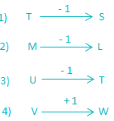→ In VW, W is the next alphabet from V, not previous.

Logical Reasoning - Test 8 - Question 2

### Select the odd number from the given alternatives.

Detailed Solution for Logical Reasoning - Test 8 - Question 2

→ if we observe carefully, we can see that the sum of digit of each number is 8 except 63.
2 + 6 = 8
4 + 4 = 8
6 + 3 = 9
3 + 5 = 8
Therefore, “63” is odd one among them.

Logical Reasoning - Test 8 - Question 3

### Choose the odd word from the given alternatives.

Detailed Solution for Logical Reasoning - Test 8 - Question 3

Quit India Movement, Non – Cooperation and Salt Satyagraha Movement were the movements led by Mahatama Gandhi but Bardoli Satyagraha Movement was led by Vallabhbhai Patel.

Therefore, “Bardoli Satyagraha Movement” is odd one among the given alternatives.

Logical Reasoning - Test 8 - Question 4

Select the related letters from the given alternatives.
AD : N : : BD : ?

Detailed Solution for Logical Reasoning - Test 8 - Question 4

Position of A = 1, D = 4 and N = 14 in English alphabets.
→ AD = N ⇒ 14 = 14
Similarly,
Position of B = 2, D = 4 and X = 24 in English alphabets.
→ BD = X ⇒ 24 = 24
Hence, “X” is the correct answer.

Logical Reasoning - Test 8 - Question 5

Select the related word from the given alternatives.
Copper : ductile ∷ chalk : ?

Detailed Solution for Logical Reasoning - Test 8 - Question 5

→ Ductility is the most important physical property of copper. Ductility of a material is its ability to deform when a force is applied upon it.
→ Brittleness is the physical property of chalk. Brittleness is exactly an opposite property of ductility as it is the ability of a material to break without first undergoing any kind of deformation.
→ In Brittleness, material breaks down without any deformation. Chalk material having such physical property therefore, chalk is related to brittle.

Logical Reasoning - Test 8 - Question 6

In the following question, select the related number from the given alternatives.
2/3 : 19/29 : : 8/7 : ?

Detailed Solution for Logical Reasoning - Test 8 - Question 6

;The pattern followed is
2/3 : 19/29
→ 2/3: (2 × 10 – 1)/(3 × 10 – 1)
Similarly,
8/7: (8 × 10 – 1)/(7 × 10 – 1)
Hence,  the missing term is 79/69.

Logical Reasoning - Test 8 - Question 7

A series is given with one term missing. Select the correct alternative from the given ones that will complete the series.
2.5, 13/6, 11/6, _____, 7/6

Detailed Solution for Logical Reasoning - Test 8 - Question 7

Sequence is obtained by following the rule given below: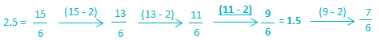Therefore, next term in series will be “1.5”

Logical Reasoning - Test 8 - Question 8

In the given series one word is missing. Select the correct alternative from the given ones that will complete the series.
Cinnaba, barcode, decibel, elastic, ______________

Detailed Solution for Logical Reasoning - Test 8 - Question 8

If we observe series carefully we can see that last two letter of each word is first two letter of next word.
CinnababarcodedecibelelasticTherefore, next term of series will start with “ic”.
In all the option only “iceberg” start with “ic”.
Therefore, “iceberg” will be next term of series.

Logical Reasoning - Test 8 - Question 9

Which set of letters when sequentially placed at the gaps in the given letter series shall complete it?
j __ l __ jk __ m __ kl __

Detailed Solution for Logical Reasoning - Test 8 - Question 9

1) jkljm ⇒ j j l k jk l m j kl m
2) kmjjj ⇒ j k l m jk j m j kl j
3) kmljm ⇒ j k l m jk l m j kl → jklm/jklm/jklm
4) mkjlm ⇒ j m l k jk j m l kl m
Hence, “kmljm” will complete the series.

Logical Reasoning - Test 8 - Question 10

How many points may be there on the face opposite to the face with 2 points?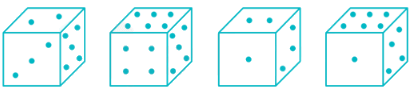Detailed Solution for Logical Reasoning - Test 8 - Question 10

From figure 1st and 2nd points 2, 3, 4 and 6 are adjacent to 5.That means 1 is opposite to 5.
As 2 and 3 are adjacent to each other, out of 4 and 6, one will be opposite to 2.
Now from the given options we can say that 6 points may be there on the face opposite to the face with 2 points.

Logical Reasoning - Test 8 - Question 11

Mithun travelled 12 kms southward, then turned left and travelled 10 kms, then turned left and travelled 12 km. How far was Mithun form the starting point?

Detailed Solution for Logical Reasoning - Test 8 - Question 11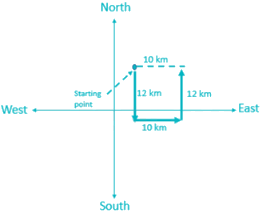Clearly, Mithun is 10 km far from starting point.

Logical Reasoning - Test 8 - Question 12

A word is represented by only one set of numbers as given in any one of the alternatives. The sets of numbers given in the alternatives are represented by two classes of alphabets as shown in the given two matrices. The columns and rows of Matrix – I are numbered from 0 to 4 and that of Matrix – II are numbered from 5 to 9. A letter from these matrices can be represented first by its row and next by its column, for example, ‘N’ can be represented by 24, 66, etc. and ‘Q’ can be represented by 42, 97 etc. Similarly, you have to identify the set for the word ‘EAGER’.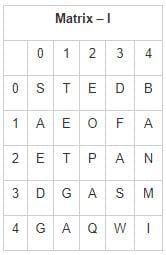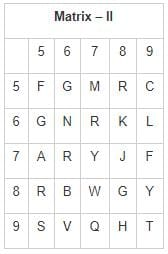Detailed Solution for Logical Reasoning - Test 8 - Question 12

1) 02, 10, 65, 11, 68 ⇒ E, A, G, E, K
2) 02, 10, 65, 87, 85 ⇒ E, A, G, W, R
3) 02, 10, 65, 11, 85 ⇒ E, A, G, E, R
4) 02, 10, 65, 59, 85 ⇒ E, A, G, C, R
Hence, “02, 10, 65, 11, 85” are set of number which represent word “EAGER”

Logical Reasoning - Test 8 - Question 13

Direction: Find which one word cannot be made from the letters of the given word.
REFRIGERATE

Detailed Solution for Logical Reasoning - Test 8 - Question 13

There are only 3 ‘E’ in word REFEREE but there required 4 ‘E’ in word REFEREE.
Therefore, option 3) is correct answer.

Logical Reasoning - Test 8 - Question 14

Which of the following diagrams indicates the best relation between snake, monkey and mammals?

Detailed Solution for Logical Reasoning - Test 8 - Question 14

→ All mammals produce milk for their babies to drink. Monkey comes under mammal category but snakes are not mammals. So option 1st is correct.

Logical Reasoning - Test 8 - Question 15

In a certain code language, "SUBSTITUTION" is written as "ITSBUSNOITUT". How is DISTRIBUTION written in that code language?

Detailed Solution for Logical Reasoning - Test 8 - Question 15

SUBSTITUTION is written as ITSBUSNOITUTSimilarly,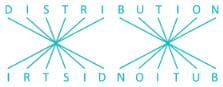DISTRIBUTION written as “IRTSIDNOITUB” in given code language.

Logical Reasoning - Test 8 - Question 16

Find the missing term in diagram shown below.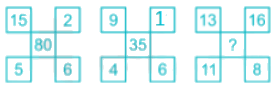Detailed Solution for Logical Reasoning - Test 8 - Question 16

In first diagram,
⇒ (15 – 5) × (6 + 2) = 10 × 8 = 80
In Second diagram,
⇒ (9 – 4) × (1 + 6) = 5 × 7 = 35
In Third diagram,
⇒ (13 – 11) × (8 + 16) = 2 × 24 = 48
Therefore, “48” is missing term.

Logical Reasoning - Test 8 - Question 17

Jais and his father has an age difference of 35 years now. After 5 years, the sum of their age is 125. What will be the age of Jais and his father after 12 years from now?

Detailed Solution for Logical Reasoning - Test 8 - Question 17

Let the present age of Jais be X years and the age of his father be Y years.
Presently, Jais and his father has an age difference of 35 years.
→ Y – X = 35. ----------- (1)
After 5 years, the sum of their ages will be 125.
→ (X + 5) + (Y + 5) = 125
→ X + Y + 10 =125
→ Y + X = 115. ----------- (2)
Adding equation (1) and (2),
Y – X + Y + X = 35 + 115
→ 2Y = 150
→ Y = 75.
Similarly, X = Y – 35 = 75 – 35 = 40.
After 12 years, Jais age is 52 (40 + 12) years and his father’s age will be 87 (75 + 12) years.
Hence, the correct answer is 52, 87.

Logical Reasoning - Test 8 - Question 18

Some equation is solved on the basis of a certain system. Find the correct answer for the unsolved equation on the basis of solved equation.
58 × 12 = 4, 37 × 96 = 5,
11 × 20 = 2, 42 × 12 =?

Detailed Solution for Logical Reasoning - Test 8 - Question 18

The given logic is as follows.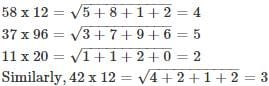Logical Reasoning - Test 8 - Question 19

Select the correct combination of mathematical signs to replace * signs and to balance the following to form an equation:
( √121 * 9) * (5 * 4) * 1

Detailed Solution for Logical Reasoning - Test 8 - Question 19

Let us first simplify the given equation:
( √121 * 9) * 5 * 4 * 1→ (11 * 9) *  5 * 4 * 1
Now we will check each option one by one:
(11 * 9) * 5 * 4 * 1→ (11 + 9) ÷ (5 × 4) = 20 ÷ (5 × 4)
Now if we take 20 ÷ (5 × 4) = 20 ÷ 20 = 1 True, thus this option can be correct.
(√121 * 9) * 5 * 4 * 1→ (11 = 9) + 5 × 4 ÷ 1 False, thus this option is wrong.
(11 * 9) * 5 * 4 * 1→ (11 – 9) × 5 + 4 = 1 False, thus this option is wrong.
(11 * 9) * 5 * 4 * 1→ (11 – 9) + 5 × 4 = 1False, thus this option is wrong.Hence possible answer is + ÷ × =.

Logical Reasoning - Test 8 - Question 20

Directions: Read the data carefully and answer the following questions.
Eight people A, B, C, D, E, F, G and H are sitting around the circular table facing towards center of it. A and B are sitting opposite to each other. F is sitting immediate right of A. There is at least one person between B and H and H is not the neighbor of F. C and D are sitting opposite to each other and none of them is sitting adjacent to F.G is not sitting opposite to F.
Question: Who is sitting opposite to H?

Detailed Solution for Logical Reasoning - Test 8 - Question 20

People are sitting around the circular table in the following way-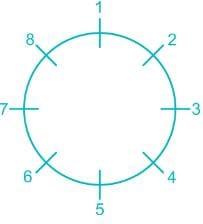All the eight positions are named from1 to 8.
Now it is told that, A and B are sitting opposite to each other and F is sitting immediate right of A.
So our arrangement will look like-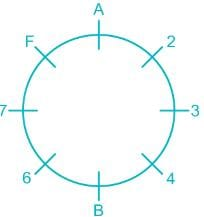Now There is at least one person between B and H and H is not the neighbor of F so H can sit at position 3, 4, or 6.
Now it is told that neither C or D is adjacent to F and C and D are opposite to each other,
Hence our arrangement will look like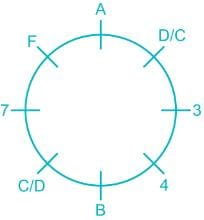So H has to take position 3.
Now it is told that G is not opposite to F then E will be opposite to F.
Then our final arrangement will look like-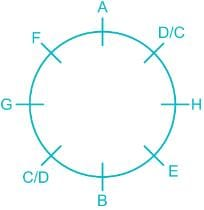Hence G is seating opposite to H.

Logical Reasoning - Test 8 - Question 21

A piece of paper is folded and cut as shown below in the question figures. From the given answer figures, indicate how it will appear when opened.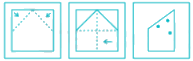Detailed Solution for Logical Reasoning - Test 8 - Question 21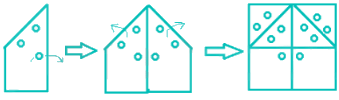One unfolding the punched paper, we get:
Thus option 3 is the correct option.

Logical Reasoning - Test 8 - Question 22

Which answer figure will complete the pattern in the question figure?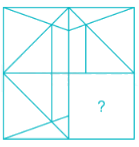Detailed Solution for Logical Reasoning - Test 8 - Question 22

The missing part is option 1 as shown below.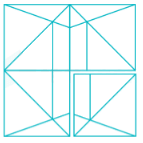Logical Reasoning - Test 8 - Question 23

If the mirror is placed on the line AB, then which of the answer figure is the right image of the given figure?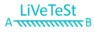Detailed Solution for Logical Reasoning - Test 8 - Question 23

Here, the correct mirror image of above figure will be,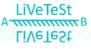Hence, the correct option is 4.

Logical Reasoning - Test 8 - Question 24

Directions: In the question below are three statements followed by three conclusions numbered I, II and III. You have to take the three given statement to be true even if they seem to be at variance from commonly known facts and then decide which of the given conclusion logically follows from the three given statements, disregarding commonly known facts.
Statements:
Some rivers are hills.
No hills are taxi.
All taxi are buses.
Conclusions:
I. Some buses are rivers.
II. Some rivers are definitely not taxi.
III. No bus is river.

Detailed Solution for Logical Reasoning - Test 8 - Question 24

From the statements, the least possible Venn diagram can be drawn as below: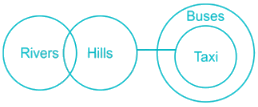Conclusions:
I. Some buses are rivers → Its possible but not definitely true, hence false.
II. Some rivers are definitely not taxis →Some rivers are hills and no hill is taxi, so that much portion of river which is hill will never be taxi. Hence true.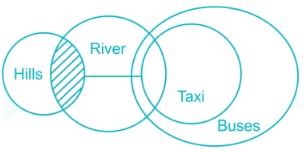III. No bus is river → Its possible but not definitely true.
Hence, only conclusion II follows.
But, since I and III make complementary pair hence, either I or III also follow.
So, conclusion II and either I or III follow.
Therefore, none of these is the correct option as there is no such option like conclusion II and either I or III follows.

Logical Reasoning - Test 8 - Question 25

In the following question, two statements are given each followed by two conclusions I and II. You have to consider the statements to be true even if they seem to be at variance from commonly known facts. You have to decide which of the given conclusions, if any, follows from the given statements.
Statement:
(I) Women generally prefer window shopping.
(II) Males generally are not fond of window shopping and they feel that it is a sheer waste of time.
Conclusions:
(I) Shopping behavior is different for males and females.
(II) If males go for window shopping, they don't utilize much time in selecting a product.

Detailed Solution for Logical Reasoning - Test 8 - Question 25

As in statement it is mentioned that Women prefer Window shopping and Males does not prefer. Hence, conclusion (I) follows as shopping behaviour is different for males and female.
But conclusion (II) does not follow as in statement nothing is mentioned if males go for windows shopping, they don’t utilize much time in selecting a product.
Hence, only conclusion I follows.

## SSC CGL Tier 1 Mock Test Series

42 docs|103 tests
 Use Code STAYHOME200 and get INR 200 additional OFF Use Coupon Code
Information about Logical Reasoning - Test 8 Page
In this test you can find the Exam questions for Logical Reasoning - Test 8 solved & explained in the simplest way possible. Besides giving Questions and answers for Logical Reasoning - Test 8, EduRev gives you an ample number of Online tests for practice

## SSC CGL Tier 1 Mock Test Series

42 docs|103 tests